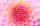# Quadratic equation

Find the roots of the quadratic equation: 3x2-4x + (-4) = 0.

Result

x1 =  2
x2 =  -0.667

#### Solution:Checkout calculation with our calculator of quadratic equations.Leave us a comment of example and its solution (i.e. if it is still somewhat unclear...):

Showing 0 comments:Be the first to comment!#### To solve this verbal math problem are needed these knowledge from mathematics:

Looking for help with calculating roots of a quadratic equation?

## Next similar examples:

1. RootsDetermine the quadratic equation absolute coefficient q, that the equation has a real double root and the root x calculate: ?
2. DiscriminantDetermine the discriminant of the equation: ?
3. EquationEquation ? has one root x1 = 8. Determine the coefficient b and the second root x2.
4. CombinationsHow many elements can form six times more combinations fourth class than combination of the second class?
5. Quadratic function 2Which of the points belong function f:y= 2x2- 3x + 1 : A(-2, 15) B (3,10) C (1,4)
6. Solve 3Solve quadratic equation: (6n+1) (4n-1) = 3n2
7. CombinationsFrom how many elements we can create 990 combinations 2nd class without repeating?
8. Variations 4/2Determine the number of items when the count of variations of fourth class without repeating is 600 times larger than the count of variations of second class without repetition.
9. 2nd class combinationsFrom how many elements you can create 4560 combinations of the second class?
10. Square root 2If the square root of 3m2 +22 and -x = 0, and x=7, what is m?
11. Variation equationSolve combinatorics equation: V(2, x+8)=72
12. Reciprocal equation 2Solve this equation: x + 5/x - 6 = 4/11
13. SeatsSeats in the sport hall are organized so that each subsequent row has five more seats. First has 10 seats. How many seats are: a) in the eighth row b) in the eighteenth row
14. Sequence 3Write the first 5 members of an arithmetic sequence: a4=-35, a11=-105.
15. Theorem proveWe want to prove the sentense: If the natural number n is divisible by six, then n is divisible by three. From what assumption we started?
16. Median and modusRadka made 50 throws with a dice. The table saw fit individual dice's wall frequency: Wall Number: 1 2 3 4 5 6 frequency: 8 7 5 11 6 13 Calculate the modus and median of the wall numbers that Radka fell.
17. Calculation of CNCalculate: ?